## 20141024

### Physics presentation: rotational dynamics¡Adios, Sapito! In this iconic cinematic moment from Raiders of the Lost Ark (Lucasfilm, Paramount Pictures, 1981), Indiana Jones outruns a gigantic round boulder. Somehow I don't think the principle of energy conservation was on his mind at the moment, but it ought to have been.Regardless of Dr. Jones having majored in archaeology instead of physics, we'll be pondering the inclusion of rotational motion into energy conservation.First, let's define some rotational parameters.Angular speed (denoted by lower-case Greek letter ω) measures how fast an object rotates, using units of radians per second, where there are 2π radians per revolution. If an object is fixed such that rotates in place, then this is the only parameter necessary to describe its motion.If an object rolls along a surface without slipping, then it will simultaneously travel with a translational speed v while rotating with an angular speed ω.A rolling object's translational speed v and angular speed ω are constrained via the "rolling without slipping" condition v = r·ω, so an object with a radius r that has a slow or fast translational speed must also be rolling with a corresponding slow or fast angular speed. (Note the peculiar nature of the radians unit, where it conveniently disappears on the right-hand side of the equation such that v results in units of meters per second.)

Here two objects with different radii have the same translational speed v, but from the v = r·ω condition, the larger r radius object has a slower ω angular speed as it rolls, while the smaller r radius object has a faster ω angular speed in order to keep up.Similar to how translational speed v and angular speed ω are related, there is a relationship between the mass M and the rotational inertia I of an object. Whereas an object's mass is a measure of how easy or difficult it is to change its translational motion, an object's rotational inertia (also known in more obscure calculus terminology as the "moment of inertia") measures the distribution of mass about its axis that makes it easy or difficult to change its rotational motion. The rotational inertia depends on the mass, size, and configuration of the object, and the appropriate expressions for rotational inertia should be used for each type of rotating object.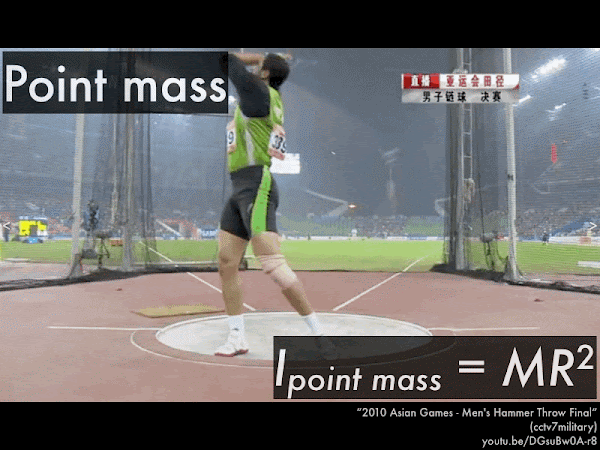The simplest rotating object is a point mass M swung around in a circle of radius R; its moment of inertia is given by Ipoint mass = M·R2. (Video link: "2010 Asian Games - Men's Hammer Throw Final.")The moment of inertia of a more complicated set of poi balls (two oil-soaked bundles at ends of chains) is simply twice the moment of inertia of a single point mass. This is the idea behind finding the moment of inertia of complex objects--merely the sum of the individual moment of inertia for its components. (Video link: "Ronan McLoughlin Fire Poi Slow motion (HD).")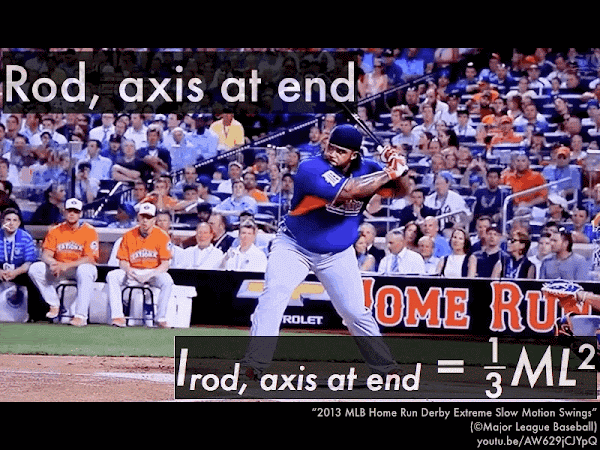Likewise for a uniform rod of mass M and length L that is swung around at one end, its moment of inertia is given by Irod, at end = (1/3)·M·R2. (The numerical factor of (1/3) for this object comes from calculus, but for the purposes of this course these numerical factors are merely to be looked up for each different type of object as needed.) (Video link: "2013 MLB Home Run Derby Extreme Slow Motion Swings.")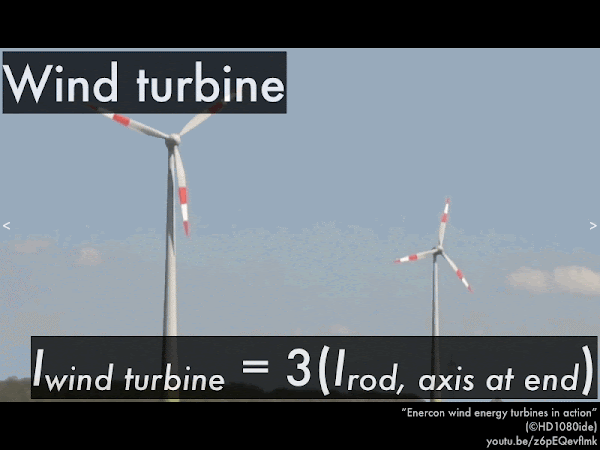The moment of inertia of a three-bladed wind turbine--which can be thought of as a set of three uniform rods swung at their ends--is merely three times the individual moment of inertia of a single uniform rod. You can always look up the moment of inertia of simple objects, and for more complex objects merely add together the moment of inertia of each of its components. (Video link: "Enercon wind energy turbines in action.")Second, incorporating rotational motion into energy conservation.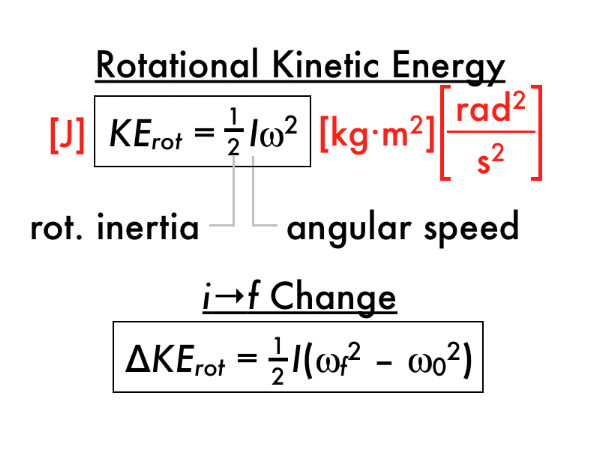Rotational kinetic energy KErot is the energy of rotational motion. Rotational kinetic energy depends on the rotational inertia I and the square of the angular speed ω of the object, and the resulting units of kg·m2/s2 are also expressed as joules (once the peculiar units of rad2 disappear). A object that is not rotating has no rotational kinetic energy, and the faster an object rotates, the more rotational kinetic energy it has.

Instead of finding out how much rotational kinetic energy KErot an object has, often we are more concerned with its initial-to-final change KErot, which is the final amount of rotational kinetic energy minus the initial amount of rotational kinetic energy. Notice how the common factors of (1/2) and rotational inertia I (which is presumed to be constant) are pulled out, leaving a "difference of squares" for the final and initial angular speeds in the parenthesis.For an object with both translational motion and rotational motion, it will have both translational kinetic energy and rotational kinetic energy. For the rider on this Solowheel, the translational kinetic energy KEtr will be given by (1/2)·(Mrider + mwheelv2, and the rotational kinetic energy KErot will be given by (1/2)·Iwheel·ω2. (Video link: "Riding the Solowheel to work - every days obstacle course.")

Note that the two kinetic energy expressions are not unrelated as the Solowheel rolls without slipping, such that the constraint v = r·ω can be used to connect the translational speed v in the translational kinetic energy expression with the angular speed ω in the rotational kinetic energy expression. We'll demonstrate this connection in some examples of objects that roll without slipping during lecture.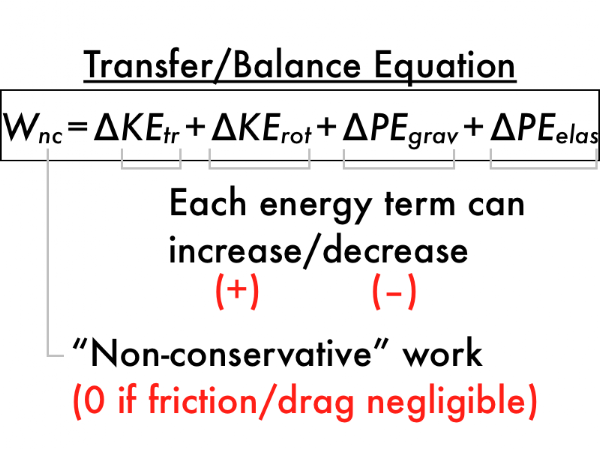Now let's incorporate rotational kinetic energy into the total energy conservation equation, which shows how the transfer of energy (as work is done by non-conservative forces acting on or against the motion of the object) causes changes in any or all of the four known mechanical energy forms on the right-hand side of the equation.

As discussed previously, in the idealized case that there are no non-conservative forces (such as friction, drag, or from external sources), then the left-hand size of this equation would be zero. Then the individual energy terms on the right-hand side of this equation can then trade and balance amongst themselves, instead of with the outside world.

So now let's see how this more comprehensive transfer/balance equation can be applied to idealized situations where friction and drag are negligible.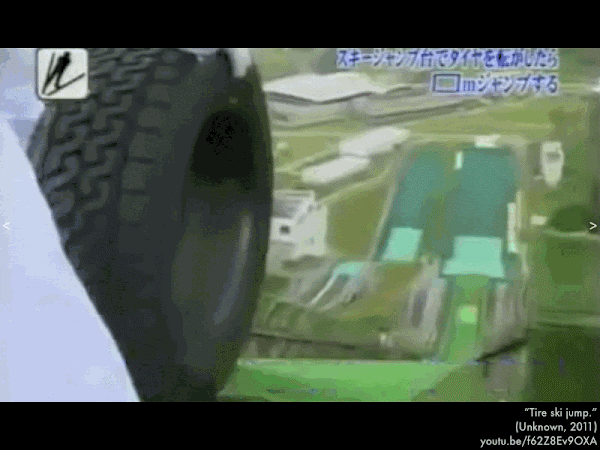A tire is released from rest at the top of a ramp, and it rolls without slipping to reach the bottom of the ramp, where it then travels upwards off of a smaller ramp, where it reaches the top of its trajectory at a height lower than its initial starting point. And this was done for science. And must-see television. And now endures as a pervasive internet meme. (Video link: "Tire ski jump.")From starting at the top of the ramp, down to the bottom of the ramp, the translational speed of the tire increases (from zero to a fast speed moving along the ramp), and since translational kinetic energy depends on the square of the tire's speed, as the tire's speed increases, its translational kinetic energy increases. (If you calculated the change in translational kinetic energy you would get a positive value, which is consistent with an increase.)

From starting at the top of the ramp, down to the bottom of the ramp, the vertical height decreases from a higher elevation to a lower elevation, and since gravitational potential energy depends on height, as the tire's height decreases, its gravitational potential energy decreases. (If you calculated the change in gravitational potential energy you would get a negative value, which is consistent with a decrease.)

Now let's consider the new type of energy introduced in this section--rotational kinetic energy. From starting at the top of the ramp, down to the bottom of the ramp, the rotational speed of the tire increases (from zero to quickly rolling along the ramp), and since rotational kinetic energy depends on the square of the tire's rotational speed, as the tire's rotational speed increases, its rotational kinetic energy increases. (If you calculated the change in rotational kinetic energy you would get a positive value, which is consistent with an increase.)

Since we are assuming friction and drag are negligible, then there is no non-conservative work done on the tire, so the left-hand side of the transfer/balance equation is zero:

Wtr = ∆KEtr + ∆PEgrav + ∆PEelas + ∆KErot,

0 = ∆KEtr + ∆PEgrav + ∆PEelas + ∆KErot,

and since there are no changes in elastic potential energy while the tire rolls down the ramp, we are only left with the changes in the translational kinetic energy, gravitational potential energy, and rotational kinetic energy terms:

0 = ∆KEtr + ∆PEgrav + 0 + ∆KErot,

0 = ∆KEtr + ∆PEgrav + ∆KErot,

0 = (+) + (–) + (+).

Now we can see that the amount that the tire's gravitational potential energy decreases (where ∆PEgrav is negative) must be directly related to the amounts that the tire's translational kinetic energy and rotational kinetic energy increase (where ∆KEtr and ∆KErot are both positive), in order to equal the zero on the left-hand side of the equation. So gravitational potential energy "feeds" (or is "transferred to") both translational kinetic energy and rotational kinetic energy during this process.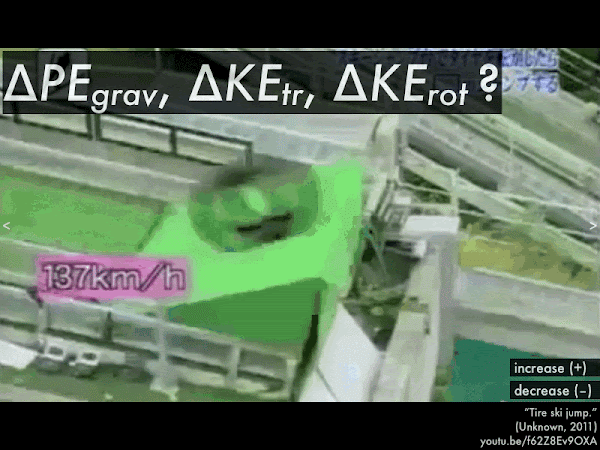For the subsequent part of this stunt, from just as it leaves the upwards lip of the ramp, to reaching the highest point of its trajectory, does the tire's translational kinetic energy increase or decrease? Does its rotational kinetic energy increase or decrease? Does its gravitational potential energy increase or decrease?

Assuming that there is no friction/drag (such that we can neglect non-conservative work), which energy system experienced a greater amount of change (whether increase or decrease) for this process: translational kinetic energy, rotational kinetic energy, or gravitational potential energy? (Note that since there is no drag, the tire just freely spins in the air, maintaining whatever rotational speed it had when it left the end of the ramp.)